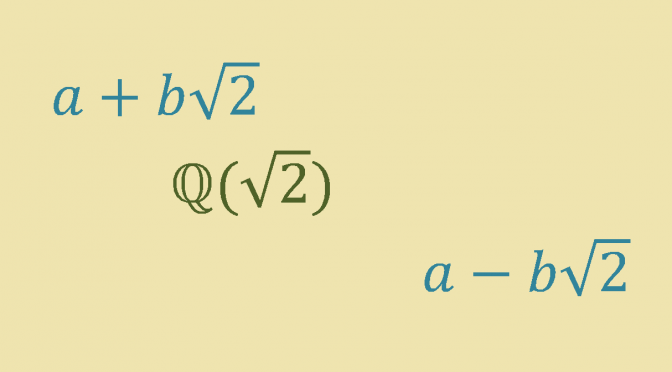# A field that can be ordered in two distinct ways

For a short reminder about ordered fields you can have a look to following post. We prove there that $$\mathbb{Q}$$ can be ordered in only one way.

That is also the case of $$\mathbb{R}$$ as $$\mathbb{R}$$ is a real-closed field. And one can prove that the only possible positive cone of a real-closed field is the subset of squares.

However $$\mathbb{Q}(\sqrt{2})$$ is a subfield of $$\mathbb{R}$$ that can be ordered in two distinct ways.

First $$\mathbb{Q}(\sqrt{2})$$ can be ordered using the induced order from $$\mathbb{R}$$ which means selecting as the positive cone the set $$P_0=\mathbb{Q}(\sqrt{2}) \cap \mathbb{R_+^*}$$. With this order, an element $$a+b\sqrt{2} \in \mathbb{Q}(\sqrt{2})$$ is positive when $$a+b\sqrt{2} \in \mathbb{R_+^*}$$.

However $$\mathbb{Q}(\sqrt{2})$$ can be ordered in a second way. The map:
$$\begin{array}{l|rcl} \varphi : & \mathbb{Q}(\sqrt{2}) & \longrightarrow & \mathbb{Q}(\sqrt{2}) \\ & a+b\sqrt{2} & \longmapsto & a-b\sqrt{2} \end{array}$$
is a field isomorphism. Consider:
$P=\{a+b\sqrt{2} ; a,b \in \mathbb{Q} \mbox{ and } a-b\sqrt{2} \in \mathbb{R_+^*}\} = \varphi^{-1}(P_0)$One can verify that $$P$$ is an ordering of $$\mathbb{Q}(\sqrt{2})$$:

• Clearly $$\mathbb{Q}(\sqrt{2})$$ is the disjoint union of $$P$$, $$\{0\}$$ and $$-P$$.
• $$P+P = \varphi^{-1}(P_0)+\varphi^{-1}(P_0)=\varphi^{-1}(P_0+P_0) \subset \varphi^{-1}(P_0)=P$$
• $$P \cdot P = \varphi^{-1}(P_0) \cdot \varphi^{-1}(P_0)=\varphi^{-1}(P_0 \cdot P_0) \subset \varphi^{-1}(P_0)=P$$

It can also be proven that there is no other ordering of $$\mathbb{Q}(\sqrt{2})$$ than the two mentioned above with:

• $$P=\{a+b\sqrt{2} ; a,b \in \mathbb{Q} \mbox{ and } a+b\sqrt{2} > 0\}$$ if $$\sqrt{2} \in P$$.
• $$P=\{a+b\sqrt{2} ; a,b \in \mathbb{Q} \mbox{ and } a-b\sqrt{2} > 0\}$$ if $$-\sqrt{2} \in P$$.

The proof is based on the equation $$(a+b\sqrt{2})(a-b\sqrt{2})=a^2-2b^2$$, the fact that $$\mathbb{Q}^*_+ \subset P$$ and a case by case analysis based on the signs of $$a$$ and $$b$$.